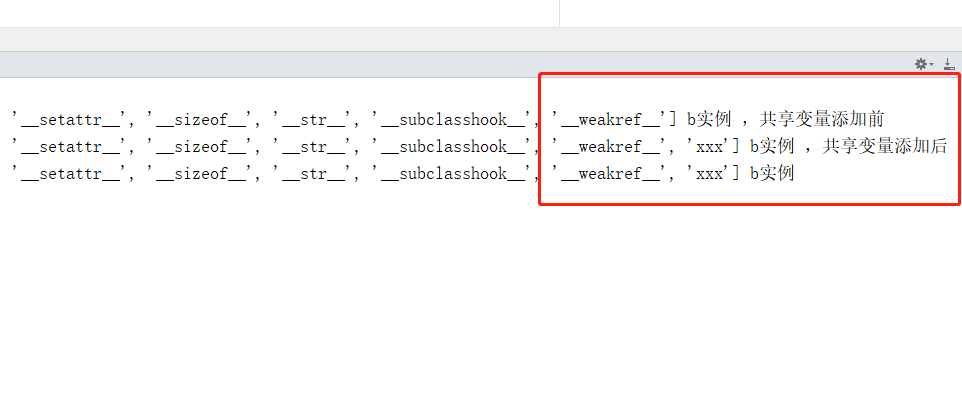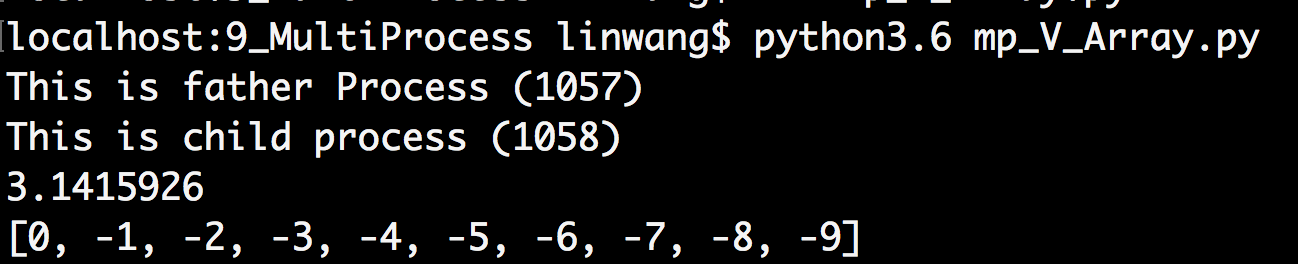• 主要介绍了Python 共享变量加锁、释放详解,文中通过示例代码介绍的非常详细，对大家的学习或者工作具有一定的参考学习价值,需要的朋友可以参考下
• 主要介绍了python共享变量操作，具有很好的参考价值，希望对大家有所帮助。一起跟随小编过来看看吧
• 主要为大家详细介绍了python多进程共享变量的相关资料，感兴趣的小伙伴们可以参考一下
• python共享变量，是不需要实例化就可以调用的， 比如类A有一个XXX的类共享变量， 直接A.xxx就可以调用 第一步：b在实例化的时候类A中没有共享变量xxx，这个时候实例b是没有这个变量 第二步...
我们以一个例子展开这个题目
问题：
python类对象A，先实例化一个A对象的实例b，接着给A对象添加一个类共享变量xxx，再实例化一个c，请问b  和c都有变量xxx吗

解答：
python类共享变量，是不需要实例化就可以调用的， 比如类A有一个XXX的类共享变量， 直接A.xxx就可以调用
第一步：b在实例化的时候类A中没有共享变量xxx，这个时候实例b是没有这个变量
第二步：给类A 添加一个类共享变量XXX， 添加完成后，会自动给类A 已生成的实例添加变量XXX  ，所以b实例在A添加共享变量XXX后，也会有变量XXX
第三步：最后实例化c的时候类A本来已经有类共享变量xxx，所以c实例也会有变量xxx

结论：实例b  c都会有变量xxx

写段代码验证下
#coding=utf-8
class A():
pass
b = A()
print(dir(b),"b实例 ，共享变量添加前")
A.xxx=10
c = A()
print(dir(b),"b实例 ，共享变量添加后")
print(dir(c),"b实例 ")
代码执行结果根据结果看， 可以看出添加类共享变量xxx之后  ，b  c 实例都有变量xxx
展开全文• 主要介绍了Python 多线程共享变量的实现示例，文中通过示例代码介绍的非常详细，对大家的学习或者工作具有一定的参考学习价值，需要的朋友们下面随着小编来一起学习学习吧
• ## Python 进程共享变量

千次阅读 2018-10-14 14:07:12
arr = Array('i', range(10)) # 定义共享内存变量, i 表示整数 p1 = Process(target=my_task, args=(arr, )) p1.start() p1.join() print(arr[:]) multiprocessing.sharedctypes 模块 除了 Value 和 ...
– Start
点击此处观看本系列配套视频。

进程有独立的内存
我们先来看一个例子。
from multiprocessing import Process

x = 1

global x
x += 1  # 在进程中修改 x
print(f'x={x}')

if __name__ == '__main__':
p1.start()
p1.join()
print(f'x={x}')


如果你认为 x 会被修改，那么你就错了。运行后，我们发现 x 的值并没有被修改，因为每个进程有独立的内存，我们无法修改其他进程中的变量。那我们一定要在进程之间共享一些数据该怎么办呢？有两种办法，一种是共享内存（Shared memory），一种是服务器进程（Server process）
共享内存（Shared memory）
Value
from multiprocessing import Process, Value

# 使用锁同步
with num.get_lock():
num.value += 1  # 在进程中修改 num

if __name__ == '__main__':
num = Value('i', 0)  # 定义共享内存变量, i 表示整数

p1.start()
p1.join()
print(f'num.value={num.value}')


Array
from multiprocessing import Process, Array

with arr.get_lock():
for i in range(len(arr)):
arr[i] = pow(arr[i], 2)

if __name__ == '__main__':
arr = Array('i', range(10))  # 定义共享内存变量, i 表示整数

p1.start()
p1.join()
print(arr[:])

multiprocessing.sharedctypes 模块
除了 Value 和 Array 外，multiprocessing.sharedctypes 模块还提供了下面的类可以在进程之间共享数据。
multiprocessing.sharedctypes.RawValue
multiprocessing.sharedctypes.RawArray

multiprocessing.sharedctypes.Value
multiprocessing.sharedctypes.Array

multiprocessing.sharedctypes.copy
multiprocessing.sharedctypes.synchronized


服务器进程（Server process）
from multiprocessing import Process, Manager

with lock:
for i in range(5):
share_dict[f'key-{i}'] = f'value-{i}'
share_list.append(f'element-{i}')

if __name__ == '__main__':
with Manager() as manager:
share_dict = manager.dict()   # 通过 manager 创建字典
share_list = manager.list()   # 通过 manager 创建列表
lock = manager.Lock()         # 通过 manager 创建锁

p1 = Process(target=my_task, args=(share_dict, share_list, lock))
p1.start()
p1.join()
print(share_dict)
print(share_list)

通过 Manager，我们不仅可以在同一台机器的不同进程之间共享数据，甚至还能不同机器的不同进程之间共享数据。感兴趣可以查阅相关文档。
– 更多参见：Python 精萃 – 声 明：转载请注明出处 – Last Updated on 2018-10-14 – Written by ShangBo on 2018-10-14 – End
展开全文• 今天小编就为大家分享一篇python多线程共享变量的使用和效率方法，具有很好的参考价值，希望对大家有所帮助。一起跟随小编过来看看吧
• 主要介绍了python使用锁访问共享变量实例解析，分享了相关代码示例，小编觉得还是挺不错的，具有一定借鉴价值，需要的朋友可以参考下
• 今天小编就为大家分享一篇浅谈python多进程共享变量Value的使用tips，具有很好的参考价值，希望对大家有所帮助。一起跟随小编过来看看吧
• 下面小编就为大家分享一篇基于python的多进程共享变量正确打开方式，具有很好的参考价值，希望对大家有所帮助。一起跟随小编过来看看吧
• Python多进程间的数据是不能共享的，那么我如果要想在Python的多进程间共享变量那么应该怎么办？ 可以使用multiprocessing中的Value和Array模块，相当于在共享内存给分配了一段内存，供给全部的进程使用。 from ...
Python多进程间的数据是不能共享的，那么我如果要想在Python的多进程间共享变量那么应该怎么办？
可以使用multiprocessing中的Value和Array模块，相当于在共享内存给分配了一段内存，供给全部的进程使用。

from multiprocessing import Process,Value,Array
import os

def f(n,a):
print('This is child process (%s)' % os.getpid())
n.value = 3.1415926
for i in range(len(a)):
a[i] = -a[i]
if __name__ == '__main__':
num = Value('d',0.0)
arr = Array('i',range(10))
print('This is father Process (%s)' % os.getpid())
p = Process(target = f,args=(num,arr))
p.start()
p.join()
print(num.value)
print(arr[:])展开全文• Python 多线程之间共享变量很简单，直接定义全局 global 变量即可。而多进程之间是相互独立的执行单元，这种方法就不可行了。 不过 Python 标准库已经给我们提供了这样的能力，使用起来也很简单。但要分两种情况来看...
Python 多线程之间共享变量很简单，直接定义全局 global 变量即可。而多进程之间是相互独立的执行单元，这种方法就不可行了。
不过 Python 标准库已经给我们提供了这样的能力，使用起来也很简单。但要分两种情况来看，一种是 Process 多进程，一种是 Pool 进程池的方式。
Process 多进程
使用 Process 定义的多进程之间共享变量可以直接使用 multiprocessing 下的 Value，Array，Queue 等，如果要共享 list，dict，可以使用强大的 Manager 模块。
import multiprocessing

def func(num):
# 共享数值型变量
# num.value = 2

# 共享数组型变量
num = 9999

if __name__ == '__main__':
# 共享数值型变量
# num = multiprocessing.Value('d', 1)
# print(num.value)

# 共享数组型变量
num = multiprocessing.Array('i', [1, 2, 3, 4, 5])
print(num[:])

p = multiprocessing.Process(target=func, args=(num,))
p.start()
p.join()

# 共享数值型变量
# print(num.value)

# 共享数组型变量
print(num[:])

Pool 进程池
进程池之间共享变量是不能使用上文方式的，因为进程池内进程关系并非父子进程，想要共享，必须使用 Manager 模块来定义。
from multiprocessing import Pool, Manager

def func(my_list, my_dict):
my_list.append(10)
my_list.append(11)
my_dict['a'] = 1
my_dict['b'] = 2

if __name__ == '__main__':
manager = Manager()
my_list = manager.list()
my_dict = manager.dict()

pool = Pool(processes=2)
for i in range(0, 2):
pool.apply_async(func, (my_list, my_dict))
pool.close()
pool.join()

print(my_list)
print(my_dict)

还有一点需要注意，在共享 list 时，像下面这样写 func 是不起作用的。
def func(my_list, my_dict):
my_list = [10, 11]
my_dict['a'] = 1
my_dict['b'] = 2

这样写相当于重新定义了一个局部变量，并没有作用到原来的 list 上，必须使用 append，extend 等方法。
参考文档：
https://blog.csdn.net/houyanhua1/article/details/78244288
展开全文多进程 编程语言
• 第7.12节 可共享Python变量.pdf
• Python 中的跨模块共享变量 一、采用general import的方法 这方法也是官方文件中FAQ中给出的方法。 在单个程序中跨模块共享信息的规范方法是创建一个特殊模块（通常称为config或cfg）。 只需在应用程序的所有模块中...
• 多线程 进程间共享变量等 1、进程间共享变量 self.val=Value('i',init_value) #进程间共享变量及列表类型 class Counter(object): def __init__(self, ini=0): self.val = Value('i', ini) self.lock = Lock...
• 首先，有5个Python文件,共享变量x存在glovar.py里面，需要在任意模块都能更改，更改后任意模块获取到的值都相同，这就是多模块文件共享一个变量。 #5个文件的路径 c:\test\main.py c:\test\show.py c:\test\test.......

# python的共享变量python 订阅